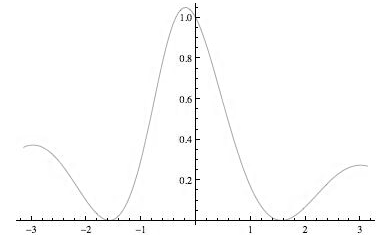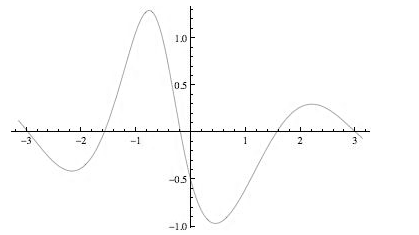# The x coordinate of the maximum and minimum points.### Single Variable Calculus: Concepts...

4th Edition
James Stewart
Publisher: Cengage Learning
ISBN: 9781337687805### Single Variable Calculus: Concepts...

4th Edition
James Stewart
Publisher: Cengage Learning
ISBN: 9781337687805

#### Solutions

Chapter 4, Problem 21RE
To determine

## To find: The x coordinate of the maximum and minimum points.

Expert Solution

The x coordinate of the maximum and minimum points are x=3,3 and x=1.6,1.6 .

### Explanation of Solution

Given information:

The function is f(x)=cos2xx2+x+1 .

Calculation:

Consider the equation.

f(x)=cos2xx2+x+1

The graph f(x) is shown figure-1Figure-1

The graph f(x) is shown figure-2Figure-2

The graph f(x) is shown figure-3Figure-3

The function has maximum value at x=3,3 and function has minimum value at x=1.6,1.6 and the inflection points for f(x) are x=2.2,0.8,0.5,2.2

### Have a homework question?

Subscribe to bartleby learn! Ask subject matter experts 30 homework questions each month. Plus, you’ll have access to millions of step-by-step textbook answers!